MEEG 342: Computer Project I

Numerical Solution of Two-Dimensional, Steady-State Temperature Distribution in a Rectangular Domain

Purpose

The purpose of this assignment is to apply the finite difference numerical technique to solve the steady-state heat conduction equation, given by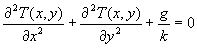(1)

where T is the temperature, x and y are the physical coordinates of the domain, g is the volumetric rate of internal heat generation (in W/m3 ), and k is the thermal conductivity.  Note that such a heat generation would, for example, be caused by passing electric current through the material.  Also note that a common mistake is not to include the heat generation rate in the proper units (recall that it represents energy per unit volume ).

Problem Definition

A two-dimensional solid (the third dimension is so long that the temperature does not vary in that direction) generates heat internally. One surfaces of the solid is insulated, two of the other surfaces are in contact with a body at constant temperatures To and T1 respectively and one surface is cooled with a coolant. We are interested in the steady state temperature distribution.

As you know, the solution of Equation (1) gives the distribution of temperature in a Cartesian domain.  This expression is a second-order, linear partial differential equation.  As in analytical solutions, we need to specify boundary conditions in order to solve the problem.  In this assignment, we will use the mixed type of boundary conditions.

Let us consider the domain shown below in Figure 1.  The block is assumed to have a height H and a width W.  A two-dimensional analysis assumes that the domain is either infinitely deep (such as a long bar, with temperature independent of the z direction), or that it is a finite block where the two surfaces parallel to the x-y plane are perfectly insulated.

The boundary conditions are: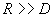at  y = 0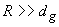at  y = H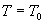at x = 0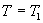at x = W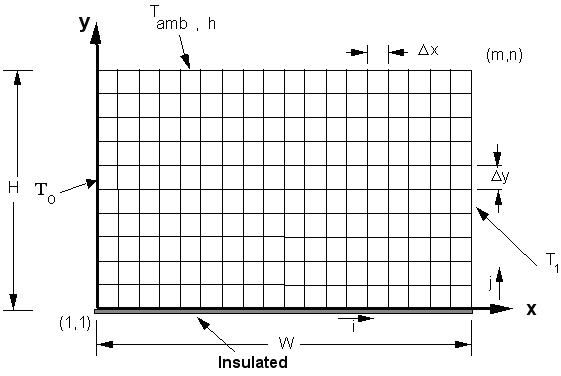Figure 1.  Problem definition

Numerical Solution Approach

Divide the domain into (m-1) parts in the x-direction and  (n-1 ) parts in the y-direction.  Discretization of the domain requires m nodes by n nodes for the mesh size, requiring a dimension statement for the temperature of   real T(m,n).  The individual nodes are numbered (i,j), where  i=1,2,3,.....m  and j=1,2,3,....n, related as shown below in Figure 2.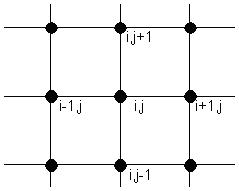Figure 2.  Grid cell notation

In addition, we will use the following for the width, height, and boundary temperatures

W = 60 cm       H =  30 cm       To= 160C        T1 = 100 C       Tamb = 20 C

SOLUTIONS

1. Temperature solution for all cases
2.  Plot the temperature solution for all cases.

Case 1a:  m=21, n=11,  k=2 W/m C ,  g=0, h=0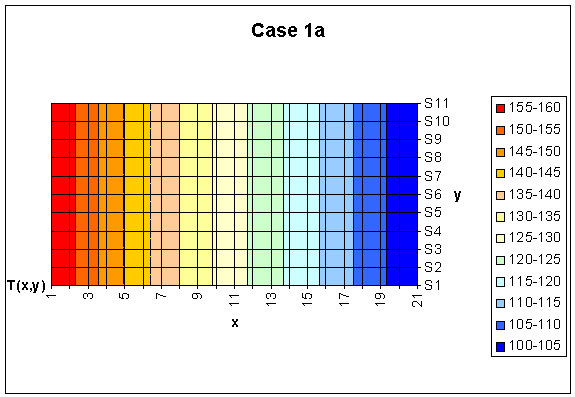Case 1b:  m=41, n=21, k=2 W/m C , g=0, h = 0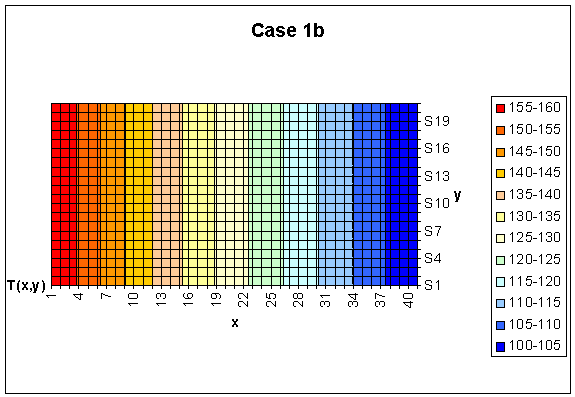Case 2a:  m=21, n=11,  k=2 W/m C ,  g=0, h = 500 W/m2 CCase 2b:  m=41, n=21, k=2 W/m C , g=0, h = 500 W/m2 C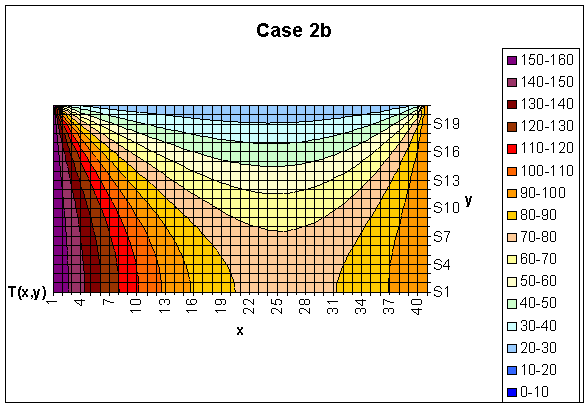Case 3a:  m=21,   n=11,   k= 50 W/m C ,  g=0.9 MW/m3 , h=0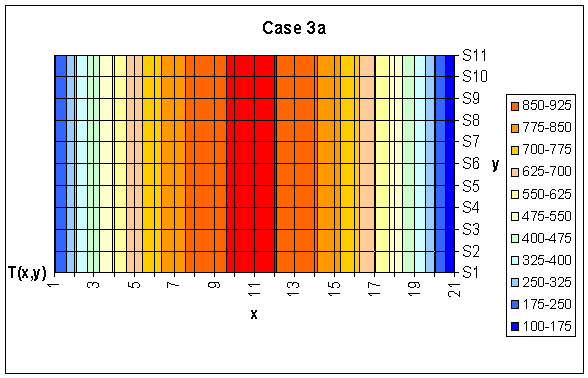Case 3b:  m=41,   n=21,   k= 50 W/m C ,  g=0.9MW/m3 , h=0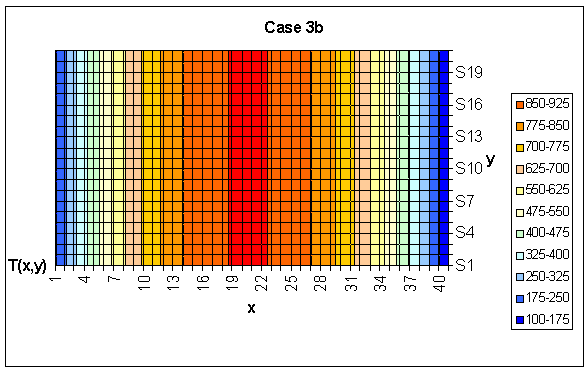Case 4a:  m=21,   n=11,   k=50 W/m C ,  g=0.9 MW/m3, h = 500 W/m2 C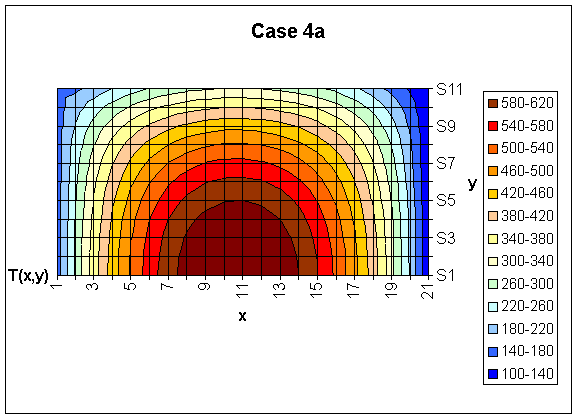Case 4b:  m=41, n=21,   k=50 W/m C ,  g=0.9 MW/m3, h = 500 W/m2 C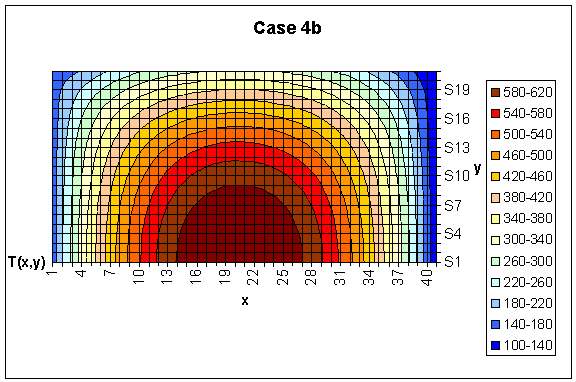1. For Case 1 as h=0 it corresponds to insulated boundary condition at the top. As the bottom is also insulated the temperature should vary only with x and should be the same along the y direction.Since T is not a function of x and y, the partial differential equation turns to an ordinary differential equation. And with g = 0;where a, b are constants. From BC’s,at x = 0 andat x = W, the analytical solution is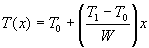With numerical values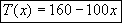Calculate q that needs to be supplied along the left wall to maintain the wall temperature at T0 analytically and compare it with the calculations of q for case 1a and 1b. Repeat the procedure at the right wall for this case. Remember for Case 1, the q supplied through the left wall should be leaving through the right wall under steady state conditions with no heat generation.

And the heat flux from left to rightWith numerical values for case 1a, at the left the heat flux for unit depth is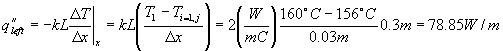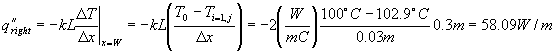For case 1b with the same formulas,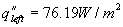and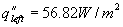For case b, the results are closer to the analytical solution.

The error for the left wall decreases from %31 to %26 and for the right wall, from %5 to %3.

Compare the temperature solution for case 1a and 1b with analytic solution for the temperature in the x-direction for steady state and no heat generation.

Case 1a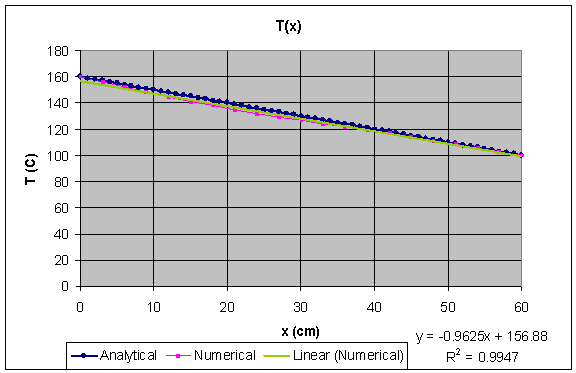Case 1b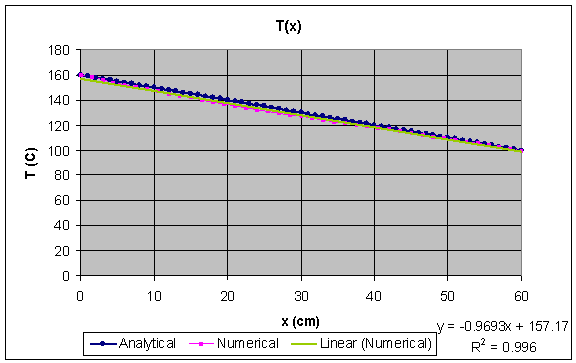As it seen from the figures, although the numerical solution is very close to the analytical one for both cases, in the second one the accuracy is better.

1. Compare the temperature solution of case 2a with 2b. Which one is more accurate? To prove your solution is correct conduct a global energy balance for case 2.

The Energy balance,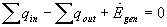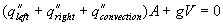and  g = 0

For unit depth and Fourier law at the left and the right wall,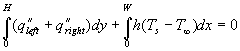For the numerical solution, one can get the heat flux by using the sum,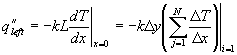Calculate the error in the energy balance for case 2a and 2b.

Case 2a: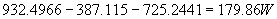Case 2b: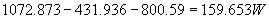In the second case, better accuracy is obtained. This is simply a result of finer mesh.

1. Case 3 is similar to case 1 except in this case there is internal heat generation.

Find the analytical solution for the temperature along the x direction and compare it with the numerical solutions obtained for cases 3a and 3b. Also calculate the q along the left and right wall and compare it with the analytic solution.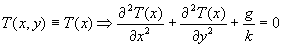Since T is not a function of x and y, the partial differential equation turns to an ordinary differential equation. And with nonzero g term;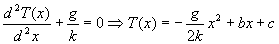where b,  c are constants. From BC’s,at x = 0 andat x = W, the constants can be found.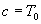and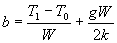then,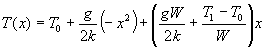With numerical values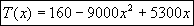And the heat flux for unit depth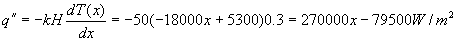At the left and the right wall the analytical solution of heat flux is,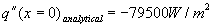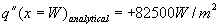With numerical values for case 3a, at the left the heat flux is,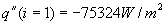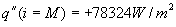And for case 3b,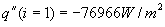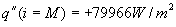The errors are found as follows, Case a @left,   Error = %5.2

Case a @right, Error = %3.1

Case b @left,   Error = %5.0

Case b @right, Error = %3.0.

For case b, the error decrease from %5 to %3.

For both cases the analytical and the numerical solution are same and the temperature distribution curves fit.1. For Case 4, compare the temperature solution of cases 4a with 4b. Conduct a   global energy balance for this case. Calculate the error in the energy balance and confirm that it goes down, as the mesh is refined.

By using the same method in question 4 for case 2 and this time adding the heat generation term to the energy balance,

The Energy balance,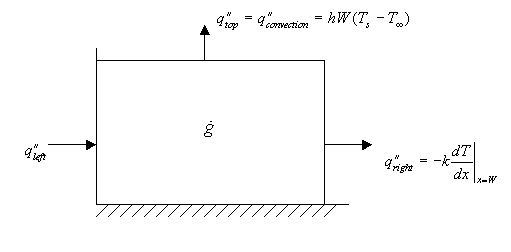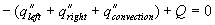For unit depth and Fourier law at the left and the right wall,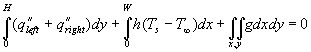For the numerical solution, one can get the heat flux by using the partial sum which is actually the discretization of the integral,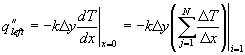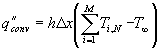Calculate the error in the energy balance for case 4a and 4b.

Case 4a: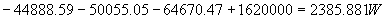Case 4b: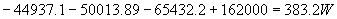The effect of finer mesh can be seen here from these results. In the second case, the result is closer to zero.

1. Discussions comparing the results of cases a with cases b, should be included. The discussion should focus on the convergence of the numerical solution and the effect of changing properties on the temperature distribution. Will any of the materials start melting?

The accuracy of a solution in finite difference method is related with the number of meshes used in the discretization. Therefore, in cases b the accuracy is better than those of in cases a.

Convergence of a numerical solution depends on the convergence accuracy defined in the sample code. Decreasing the convergence accuracy leads to more accurate results.

The effect of thermal conductivity coefficient can be seen by comparing the first two and the last two cases. When k is increased from 2 to 50, heat transfer increases. The heat generation leads to higher temperatures and higher thermal conductivity is required in order to transfer heat from the system and avoid high temperatures that might cause melting of the material.

Extra Notes…

Derivation of the equations by using finite difference method for the top boundary:The Energy balance,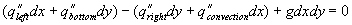With dx=dy=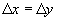and for unit depth,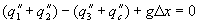Since we have half of the cell,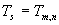in the figure above. Divide by k all terms,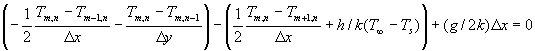Rearrange the equation by multiplying all terms with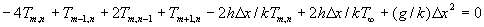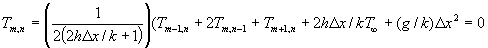Sample Code

'MEEG302 Heat Transfer

'example program for computer assignment 1

'this program calculates the temperature distribution in a simple

'rectangular domain with constant heat flux at the top and constant

'temperature at the other three sides

Sub sample()

'define constants

Const M = 21 'grid size

Const N = 11 '

Const X = 0.6 'rectangular dimensions (m)

Const Y = 0.3 '

Const g = 900000 'heat generation

Const h = 0 'convection coefficient

Const T0 = 160 'constant temperature at side : left

Const T1 = 100 'constant temperature at side : right

Const Ts = 20 'surrounding temp

Const EPS = 0.01 'convergence accuracy

Const k = 50 'conduction coeff

'define arrays and variables

Dim Tnew(M, N), T(M, N) As Single 'temperatures

Dim isConverged As Boolean 'flag

Dim dx, dy As Single 'delta x and delta y

'initialize variables

dx = X / (M - 1)

dy = Y / (N - 1)

'initialize the temperature array

For i = 1 To M

For j = 1 To N

T(i, j) = 125

Tnew(i, j) = 125

Next j

Next i

'**** main loop

'this loop keeps recalculating the temperature field until

'it has converged

Do

'Tnew() holds the newly calculated temperatures

'T() holds the values from the last iteration

'top boundary (convection)

For i = 2 To M - 1

j = N

Tnew(i, j) = (1 / (2 * (2 + h * dx / k))) * (2 * T(i, j - 1) + T(i - 1, j) + T(i + 1, j) + 2 * h * dx * Ts / k + g * dx * dy / k)

Next i

'left boundary (constant temp)

For j = 1 To N

i = 1

Tnew(i, j) = T0

Next j

'right boundary (constant temp)

For j = 1 To N

i = M

Tnew(i, j) = T1

Next j

'bottom boundary (constant temp)

For i = 2 To M - 1

j = 1

Tnew(i, j) = (1 / 4) * (2 * T(i, j + 1) + T(i - 1, j) + T(i + 1, j) + g * dx * dy / k)

Next i

'interior nodes

For i = 2 To M - 1

For j = 2 To N - 1

Tnew(i, j) = (1 / 4) * (T(i - 1, j) + T(i + 1, j) + T(i, j - 1) + T(i, j + 1) + g * dx * dy / k)

Next j

Next i

'check to see if the temperatures have converged

isConverged = True

For i = 1 To M

For j = 1 To N

If Abs(T(i, j) - Tnew(i, j)) > EPS Then isConverged = False

Next j

Next i

'copy new temperatures to old temperatures and display on the worksheet

For i = 1 To M

For j = 1 To N

'this command displays the elements of T on the worksheet

'the command is Cells(row,column)

'because Excel counts rows from the top the grid is

'actually printed upside down

Cells(j + 1, i + 1) = Tnew(i, j)

'copy new temperatures to old array T

T(i, j) = Tnew(i, j)

Next j

Next i

'stop the loop if the values have converged

Loop While (isConverged = False)

End Sub